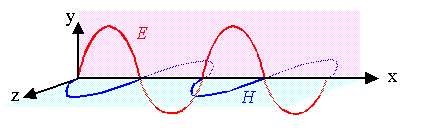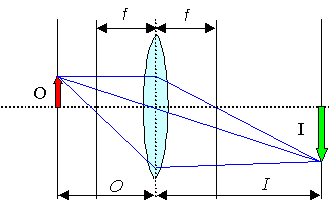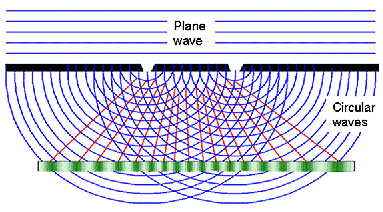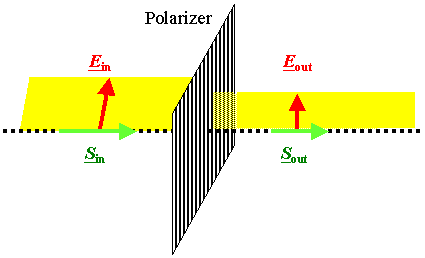### 5.1.5 Summary to: 5.1 OpticsKnow your numbers and relations for visible light!
 For the propagation of light: use the wave model For the generation and disappearance (= absorption) of light: use the photon model

 Snellius law: n = sina/sinb with a, b the angle of incidence or propagation, resp.Wavelengths: l » 400 nm - 800 nm.
lmat = l0/n.Frequency: n» 10 15 Hz.Index of refraction: n = er½ » 1,5 - 2,5Energy E » 1,8 eV - 3,2 eV.Dispersion relation: c0 = n l0 = 3 · 108 m/s
cMat = n l0/n(l)Know yout basic equations and terminologyCoherent monochromatic plane wave E and H perpendicular and in phase

E(r,t)
H(r,t)
=   E0
H0
· exp{i(krwt)}Reflection always with "angle in" = "angle out".Refraction is the sudden "bending" or "flexing" of light beams at the interfaceDiffraction is the continous "bending" of light beams around corners; interference effects.Geometric optics
Key paramtersFocal length f and
numerical aperture NA of lenses, mirrors.Image formation by simple geometric constrationVarious aberrations (spherical. chromatic, astigmatism, coma, ...) limit performance.Wave optics
Huygens principle: and interferenceUltimate limit to resolution

dmin  »  l
2NAKnow your basic types of waves:(Running, coherent, monochromatic) plane wave.Standing waves = superposition of plane waves.Incoherent, multichromatic real wavesRelation s between electrical field E, magnetic field H and Poynting vector (energy flow vector) S = E × H
Welect   =  e0 · E2
2
Wmag   =  m0 · H2
2
[Welect; magn]   =  [Ws m–3]
E0   = æ ç è ö ÷ ø

<S>  =  E0H0
2
=  E02
Zw

This equation links energy flow (easy in photon picture) to field strength in wave picture.Zw = wave impedance of the medium.
Zw(vacuum) = 376,7 WPolarization = key to "advanced" optics.
Simple case: linear polarization.Plane of polarization contains E-vector and S (k) vector.Any (coherent) wave is polarized but net polarization of many waves with random polarization is zero!Light intensity (µ E2) between polarizers at angle a scales with (cosa)2.General case: elliptical polarization; important are the two extremes: linear and circular polarization.For circular polarizaiton the E-vector rotates on a circle while moving "forward". This results from a superposition of two plane waves with E-vectors ar right angles and a phase difference of p/2.Technically important (3-dim Cinema; Lab optics)
 Questionaire Multiple Choice questions to all of 5.1